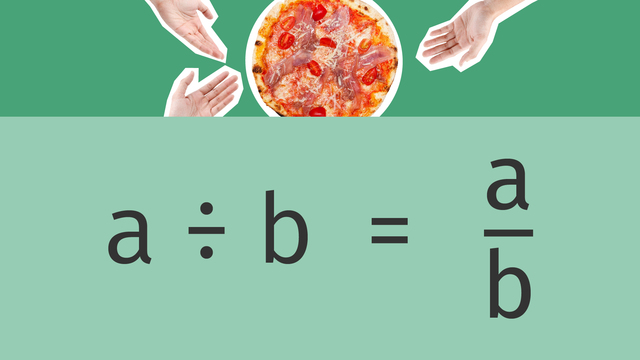# Interpreting Fractions as DivisionRating

Be the first to give a rating!
The authorsTeam Digital
Interpreting Fractions as Division
5.NF.B.3

## Basics on the topicInterpreting Fractions as Division

How are division sentences and fractions related? Learn about interpreting fractions as division with this video.

### TranscriptInterpreting Fractions as Division

Sometimes when we share things, we have less to distribute than the number we are sharing between. And that can be really tricky math to solve, right? Well, it doesn't have to be! Interpreting fractions as division. You may remember that fractions consist of two parts; the numerator and the denominator. The denominator, B, tells us how many equal parts the whole is divided into, and the numerator, A, tells us how many parts of the whole we have. Let's explore how we can rewrite a fraction as a division sentence. Imagine that you have a whole pizza, and you want to share it equally among four people. That means you need to divide the whole pizza into four equal parts. When we write this as a division sentence, it is one divided by four. This would mean that each person gets an equal part of the pizza, which can be rewritten in fraction form as one over four. This is how we can interpret a division as a fraction, and also a fraction as a division sentence! Let's take a look at another example. This time, we have three subs, and we are sharing them between five people. Let's use fraction bars to help us make sense of this one. As you may already notice, each person this time would get three fifths of a sub. Let's make sure this division works by using fraction bars. If we divide each sub into fifths, we can see that if we distribute each part equally between the five friends, we end up with three parts per person, or three-fifths. Now it's your turn! If you have two chocolate bars, and you share it among three people, what fraction of chocolate does each person get? Pause the video to solve, and press play when you are ready to see the solution. Since we are dividing two by three, each person gets two-thirds of a chocolate bar. Here is one more problem. This time, we have the fraction four-eighths. What is the division sentence that matches this fraction? Pause the video to solve, and press play when you are ready to see the solution. Four-eighths tells us that we have four whole things to share between eight, so it is four divided by eight. To summarize, the fraction A over B can be rewritten as A divided by B, and A divided by B can be rewritten as a fraction A over B. Fractions represent dividing a whole into equal parts. So now, whenever you see a fraction, think of it as a division problem, and you can make sense of fractions going forward!